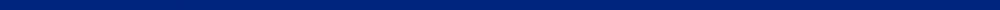CIVL 7117 - Schedule (subject to change)Date Topic TBD Introduction to Finite Elements - History and applications Chapter 2 - Definition of the stiffness matrix; applications for a simple spring element;                    and potential energy approach to derive spring element equations. Holiday - Labor Day Chapter 3 - Development of the stiffness matrix for a bar element and transformation of                    global stiffness matrix for a bar arbitrarily oriented in a plane Chapter 3 - Transformation matrix for a bar in three-dimensions; inclined or skewed supports;                    and one-dimensional elastic rod using potential energy Chapter 3 - One-dimensional elastic rod using Galerkin's method; symmetry and                    bandwidth of bar stiffness matrix SAP2000  - Truss analysis Chapter 4- Beam stiffness and example of assemblage of beam stiffness equations and                  examples using direct stiffness Chapter 4 - Distributed loadings; comparison of FE solution to exact solution;                    beam element with nodal hinge; potential energy approach to deriving beam                    equations; and Galerkin's method for deriving beam equations Chapter 5 - Two-dimensional arbitrarily oriented beam element for frames and                    rigid frame examples Chapter 5 - Two-dimensional arbitrarily oriented beam element for frames;                    rigid frame examples; and incline supports; and grid elements SAP2000 Introduction - Frame and grid analysis Chapter 6 - Development of the Plane Stress Element (CST) Chapter 6 - Development of the Plane Stress Element (CST) - Problems Fall Break Chapter 6 - Development of the Plane Stress Element (Q4 element) Chapter 7 - Practical Consideration on Modeling Mid-Term Exam Assigned Chapter 8 - Development of Linear-Strain Triangular Equations Chapter 9 - Axisymmetric Elements Chapter 9 - Axisymmetric Elements Chapter 10 - Isoparametric Formulation of the Bar and Q4 Elements Chapter 10 - Newton-Cotes and Gaussian Quadrature Chapter 10 - Isoparametric Formulations for a Linear Strain Bar; Q6, Q8, Q9, and Q12 Elements Chapter 12 - Plate Bending Elements Chapter 12 - Using SAP2000 to analyze plate/shell problem Chapter 16 - Dynamics analysis of SDOF systems Chapter 16 - Dynamics analysis using bar, beam, frames, trusses, and CST elements SAP2000 to analyze structural dynamic problems Chapter 11 - Three-Dimensional Stress Analysis using tetrahedral and hexahedral elements Chapter 11 - Three-Dimensional Stress Analysis using tetrahedral and hexahedral elements Final Exam Dues

This web site was originally developed by Charles Camp for CIVL 7117.
This site is Maintained by the Department of Civil Engineering at the University of Memphis.